### Create an Account

Home / Questions / A cylindrical tank 5 m in diameter discharges through a mild steel pipe system

# A cylindrical tank 5 m in diameter discharges through a mild steel pipe system

A cylindrical tank, 5 m in diameter, discharges through a mild steel pipe system (ε = 4.6 × 10−5 m) connected to the tank base as shown in the figure below. The drain pipe system has an equivalent length of 100 m and a diameter of 23 cm. The tank is initially filled with water to an elevation of with respect to the reference plane.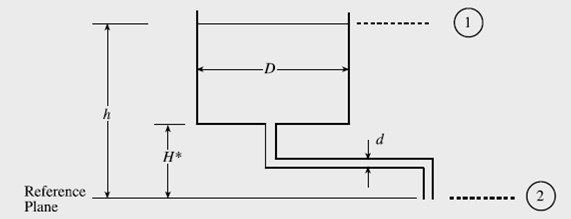a) Apply the Bernoulli equation, Eq. (5) in Problem 7.7, to the region between planes “1” and “2” and show that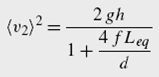where Leq is the equivalent length of the drain pipe.

b) Consider the tank as a system and show that the application of the unsteady-state macroscopic mass balance gives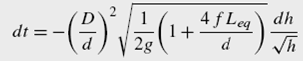Analytical integration of Eq. (2) is possible only if the friction factor is constant.

c) At any instant, note that the pressure drop in the drain pipe system is equal to ρg(hH). Use Eqs. (4.5-18)–(4.5-20) to determine as a function of liquid height in the tank. Take H =1 m, = 4 m, and the final value of as 1.5 m.

Eqs. (4.5-18)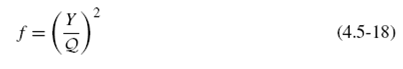Eqs. (4.5-20)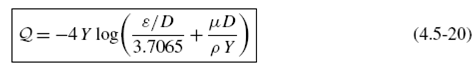d) If remains almost constant, then show that the integration of Eq. (2yields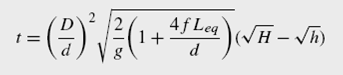Calculate the time required for to drop from 4 m to 1.5 m.

e) Plot the variations of <v2> and as a function of time on the same plot. Show that dh/dt is negligible at all times in comparison with the liquid velocity through the drain pipesystem.

View less »

Jul 25 2020 View more View LessSubscribe To Get Solution